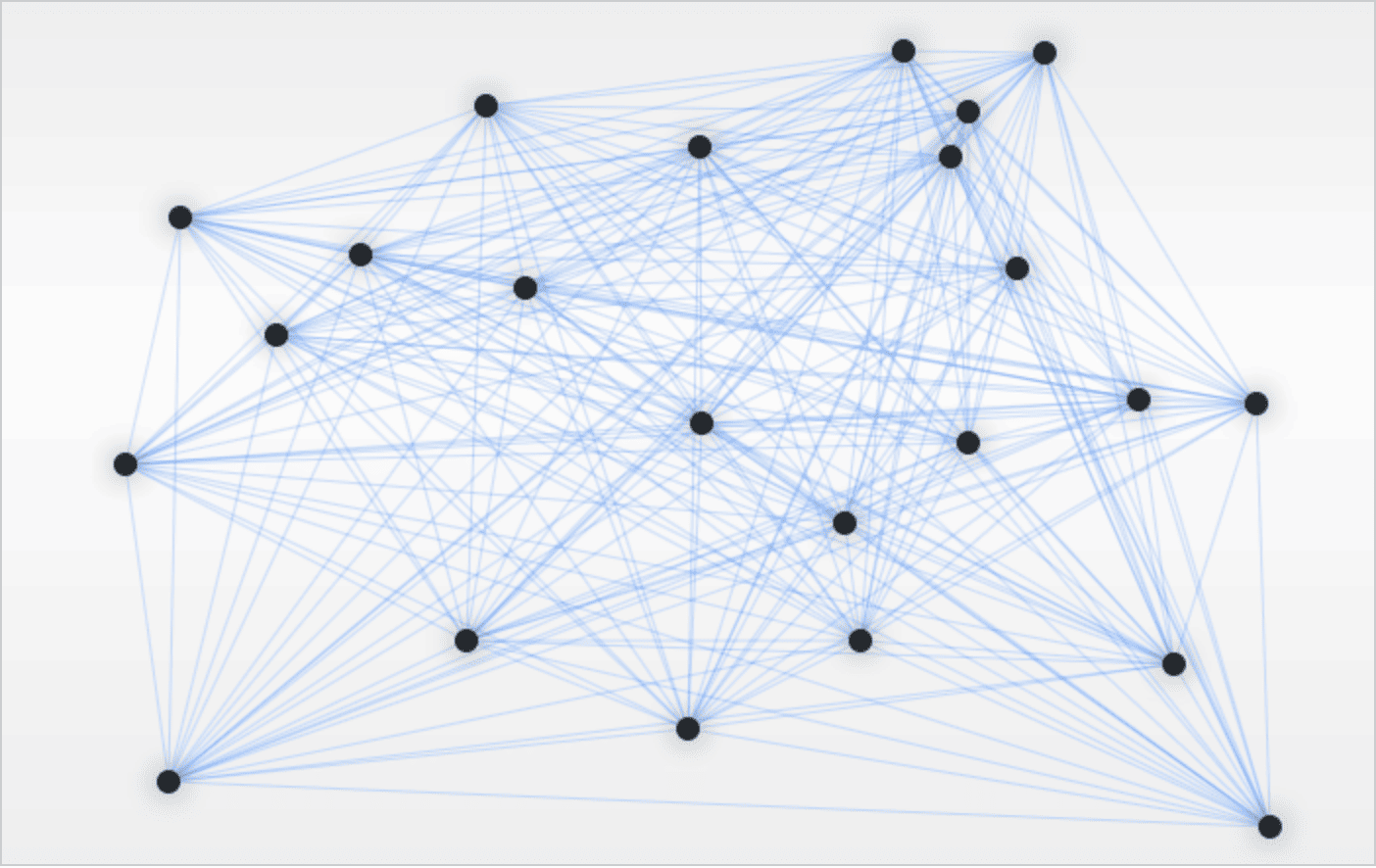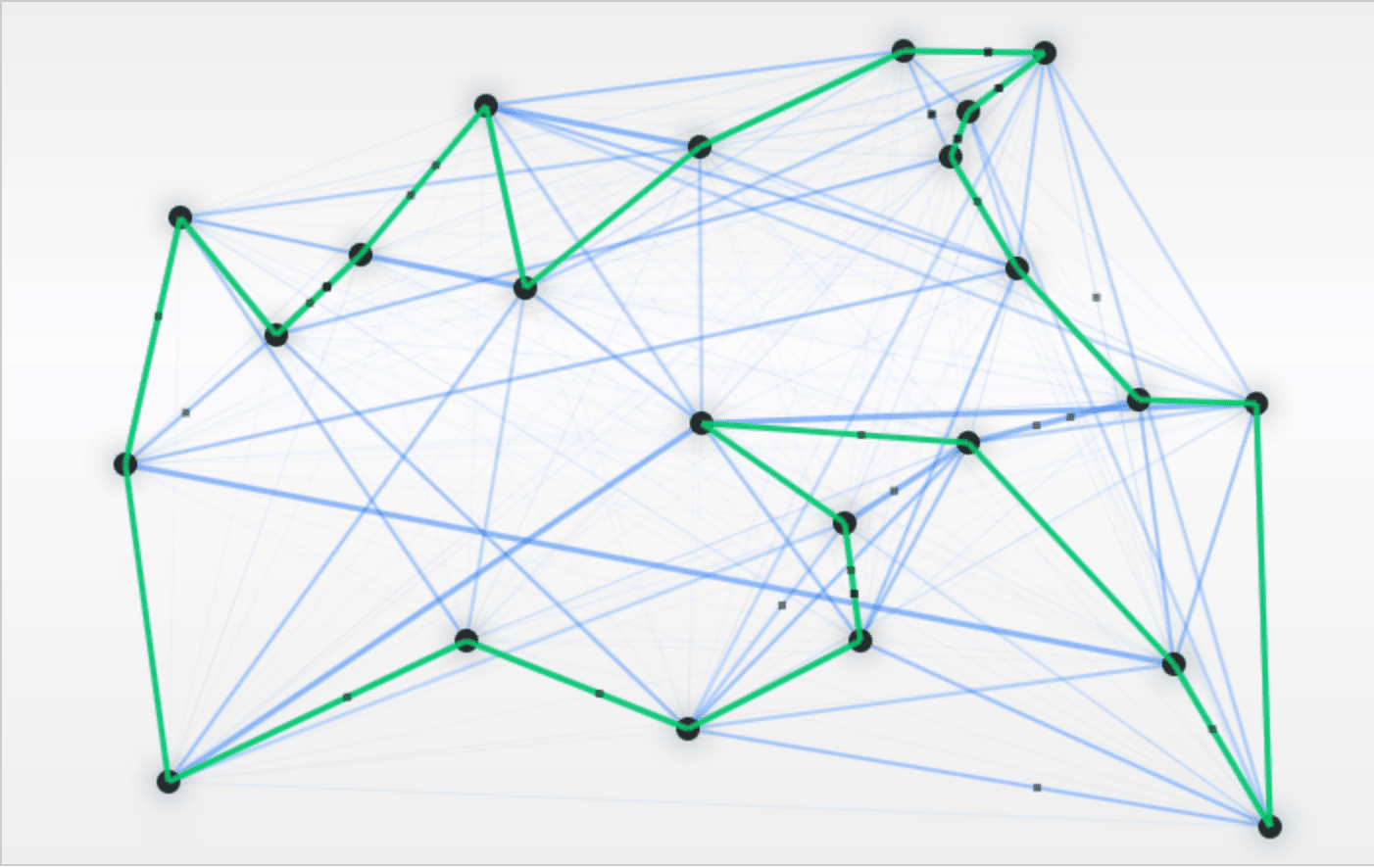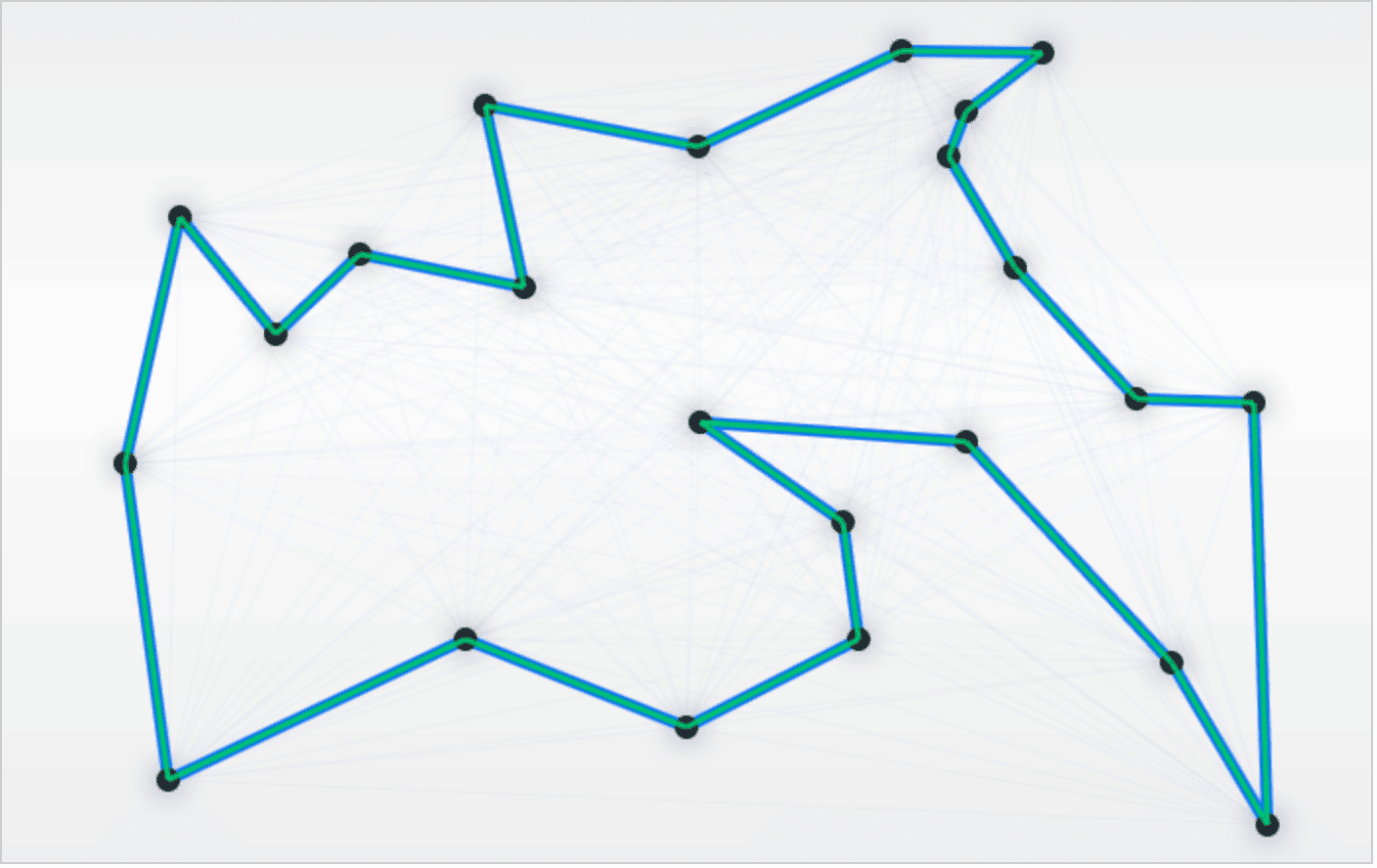We're now running the only sale of the year - our Black Friday launch. All Courses are 30% off until end-of-day today:

## 1. Introduction

The aim of this series is to explain the idea of genetic algorithms and show the most known implementations.

In this tutorial, we’ll describe the concept of the ant colony optimization (ACO), followed by the code example.

## 2. How ACO Works

ACO is a genetic algorithm inspired by an ant’s natural behavior. To fully understand the ACO algorithm, we need to get familiar with its basic concepts:

• ants use pheromones to find the shortest path between home and food source
• pheromones evaporate quickly
• ants prefer to use shorter paths with denser pheromone

Let’s show a simple example of ACO used in the Traveling Salesman Problem. In the following case, we need to find the shortest path between all nodes in the graph:Following by natural behaviors, ants will start to explore new paths during the exploration. Stronger blue color indicates the paths that are used more often than the others, whereas green color indicates the current shortest path that is found:As a result, we’ll achieve the shortest path between all nodes:The nice GUI-based tool for ACO testing can be found here.

## 3. Java Implementation

### 3.1. ACO Parameters

Let’s discuss the main parameters for the ACO algorithm, declared in the AntColonyOptimization class:

``````private double c = 1.0;
private double alpha = 1;
private double beta = 5;
private double evaporation = 0.5;
private double Q = 500;
private double antFactor = 0.8;
private double randomFactor = 0.01;``````

Parameter c indicates the original number of trails, at the start of the simulation. Furthermore, alpha controls the pheromone importance, while beta controls the distance priority. In general, the beta parameter should be greater than alpha for the best results.

Next, the evaporation variable shows the percent how much the pheromone is evaporating in every iteration, whereas Q provides information about the total amount of pheromone left on the trail by each Ant, and antFactor tells us how many ants we’ll use per city.

Finally, we need to have a little bit of randomness in our simulations, and this is covered by randomFactor.

### 3.2. Create Ants

Each Ant will be able to visit a specific city, remember all visited cities, and keep track of the trail length:

``````public void visitCity(int currentIndex, int city) {
trail[currentIndex + 1] = city;
visited[city] = true;
}

public boolean visited(int i) {
return visited[i];
}

public double trailLength(double graph[][]) {
double length = graph[trail[trailSize - 1]][trail];
for (int i = 0; i < trailSize - 1; i++) {
length += graph[trail[i]][trail[i + 1]];
}
return length;
}
``````

### 3.3. Setup Ants

At the very beginning, we need to initialize our ACO code implementation by providing trails and ants matrices:

``````graph = generateRandomMatrix(noOfCities);
numberOfCities = graph.length;
numberOfAnts = (int) (numberOfCities * antFactor);

trails = new double[numberOfCities][numberOfCities];
probabilities = new double[numberOfCities];
ants = new Ant[numberOfAnts];

Next, we need to setup the ants matrix to start with a random city:

``````public void setupAnts() {
IntStream.range(0, numberOfAnts)
.forEach(i -> {
ants.forEach(ant -> {
ant.clear();
ant.visitCity(-1, random.nextInt(numberOfCities));
});
});
currentIndex = 0;
}``````

For each iteration of the loop, we’ll perform the following operations:

``````IntStream.range(0, maxIterations).forEach(i -> {
moveAnts();
updateTrails();
updateBest();
});``````

### 3.4. Move Ants

Let’s start with the moveAnts() method. We need to choose the next city for all ants, remembering that each ant tries to follow other ants’ trails:

``````public void moveAnts() {
IntStream.range(currentIndex, numberOfCities - 1).forEach(i -> {
ants.forEach(ant -> {
ant.visitCity(currentIndex, selectNextCity(ant));
});
currentIndex++;
});
}``````

The most important part is to properly select next city to visit. We should select the next town based on the probability logic. First, we can check if Ant should visit a random city:

``````int t = random.nextInt(numberOfCities - currentIndex);
if (random.nextDouble() < randomFactor) {
OptionalInt cityIndex = IntStream.range(0, numberOfCities)
.filter(i -> i == t && !ant.visited(i))
.findFirst();
if (cityIndex.isPresent()) {
return cityIndex.getAsInt();
}
}``````

If we didn’t select any random city, we need to calculate probabilities to select the next city, remembering that ants prefer to follow stronger and shorter trails. We can do this by storing the probability of moving to each city in the array:

``````public void calculateProbabilities(Ant ant) {
int i = ant.trail[currentIndex];
double pheromone = 0.0;
for (int l = 0; l < numberOfCities; l++) {
if (!ant.visited(l)){
pheromone
+= Math.pow(trails[i][l], alpha) * Math.pow(1.0 / graph[i][l], beta);
}
}
for (int j = 0; j < numberOfCities; j++) {
if (ant.visited(j)) {
probabilities[j] = 0.0;
} else {
double numerator
= Math.pow(trails[i][j], alpha) * Math.pow(1.0 / graph[i][j], beta);
probabilities[j] = numerator / pheromone;
}
}
}
``````

After we calculate probabilities, we can decide to which city to go to by using:

``````double r = random.nextDouble();
double total = 0;
for (int i = 0; i < numberOfCities; i++) {
total += probabilities[i];
if (total >= r) {
return i;
}
}``````

### 3.5. Update Trails

In this step, we should update trails and the left pheromone:

``````public void updateTrails() {
for (int i = 0; i < numberOfCities; i++) {
for (int j = 0; j < numberOfCities; j++) {
trails[i][j] *= evaporation;
}
}
for (Ant a : ants) {
double contribution = Q / a.trailLength(graph);
for (int i = 0; i < numberOfCities - 1; i++) {
trails[a.trail[i]][a.trail[i + 1]] += contribution;
}
trails[a.trail[numberOfCities - 1]][a.trail] += contribution;
}
}``````

### 3.6. Update the Best Solution

This is the last step of each iteration. We need to update the best solution in order to keep the reference to it:

``````private void updateBest() {
if (bestTourOrder == null) {
bestTourOrder = ants.trail;
bestTourLength = ants.trailLength(graph);
}
for (Ant a : ants) {
if (a.trailLength(graph) < bestTourLength) {
bestTourLength = a.trailLength(graph);
bestTourOrder = a.trail.clone();
}
}
}``````

After all iterations, the final result will indicate the best path found by ACO. Please note that by increasing the number of cities, the probability of finding the shortest path decreases.

## 4. Conclusion

This tutorial introduces the Ant Colony Optimization algorithm. You can learn about genetic algorithms without any previous knowledge of this area, having only basic computer programming skills.

The complete source code for the code snippets in this tutorial is available in the GitHub project.

For all articles in the series, including other examples of genetic algorithms, check out the following links:

We're now running the only sale of the year - our Black Friday launch. All Courses are 30% off until end-of-day today: